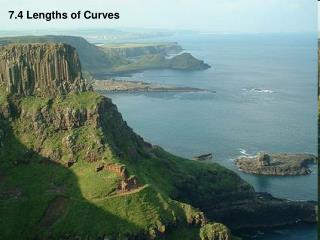DownloadDownload Presentation7.4 Lengths of Curves

# 7.4 Lengths of Curves

Download Presentation## 7.4 Lengths of Curves

- - - - - - - - - - - - - - - - - - - - - - - - - - - E N D - - - - - - - - - - - - - - - - - - - - - - - - - - -
##### Presentation Transcript

1. 7.4 Lengths of Curves

2. 2+x csc x 1 0 -1

3. Length of Curve (Cartesian) Lengths of Curves: If we want to approximate the length of a curve, over a short distance we could measure a straight line. By the pythagorean theorem: We need to get dx out from under the radical.

4. Now what? This doesn’t fit any formula, and we started with a pretty simple example! The TI-89 gets: Example:

5. If we check the length of a straight line: Example: The curve should be a little longer than the straight line, so our answer seems reasonable.

6. Y Y F4 ENTER ENTER ENTER STO Example: You may want to let the calculator find the derivative too: Important: You must delete the variable y when you are done! 4

7. Example:

8. X ENTER STO If you have an equation that is easier to solve for x than for y, the length of the curve can be found the same way. Notice that x and y are reversed.

9. Y X F4 ENTER Don’t forget to clear the x and y variables when you are done! 4 p

10. Getting Around a Corner Find the length of the curve y = x2 – 4|x| - x from x = -4 to x=4.

11. Ch 7.4 Surface Area

12. r Surface Area about x-axis (Cartesian): To rotate about the y-axis, just reverse x and y in the formula! Surface Area: Consider a curve rotated about the x-axis: The surface area of this band is: The radius is the y-value of the function, so the whole area is given by: This is the same ds that we had in the “length of curve” formula, so the formula becomes:

13. Example: Rotate about the y-axis.

14. Example: Rotate about the y-axis.

15. From geometry: Example: Rotate about the y-axis.

16. Y ENTER ENTER STO rotated about x-axis. Example:

17. Y ENTER ENTER STO Check: rotated about x-axis. Example:

18. Y X F4 ENTER Don’t forget to clear the x and y variables when you are done! Once again … 4 p

19. Find the area of the surface formed by revolving the graph of f(x) = x3 on the interval [0,1] about the x axis.

20. Find the area of the surface formed by revolving the graph of f(x) = x2 on the interval [0,√2] about the y axis.